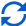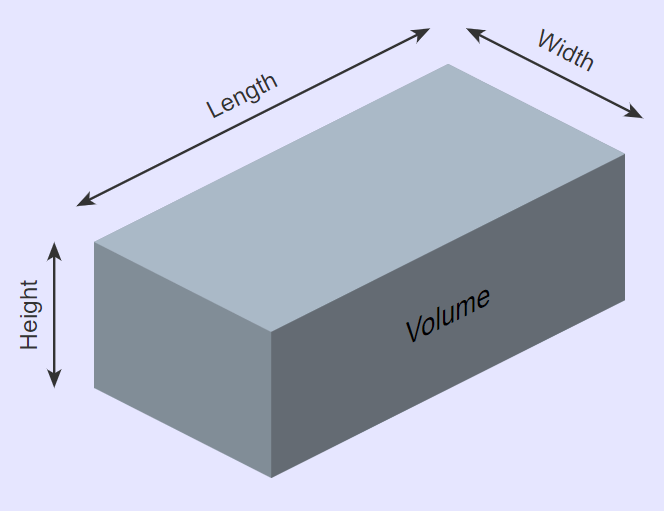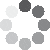Enter parameters
Enter parameters
LENGTH, WIDTH & DEPTH
Length:
ft
• inches (in)
• feet (ft)
• yards (yd)
• centimeters (cm)
• meters (m)
Width:
ft
• inches (in)
• feet (ft)
• yards (yd)
• centimeters (cm)
• meters (m)
Height:
in
• inches (in)
• feet (ft)
• yards (yd)
• centimeters (cm)
• meters (m)
Price per unit of volume:
yd³
• cubic yards (yd³)
• cubic feet (ft³)
• cubic inches (in³)
• cubic centimeters (cm³)
• cubic meters ()Result
Volume:
0
yd³
• cubic yards (yd³)
• cubic feet (ft³)
• cubic inches (in³)
• cubic centimeters (cm³)
• cubic meters ()
Cost:
$(6 votes, average: 4.33 out of 5)Loading... Similar Calculators: Real-time graphics. Make the calculations and see the changes. Embed Similar Calculators:A construction or landscaping project will often require you to first determine how much material you will need. It is important that you are able to calculate an accurate measurement, which will save you both time and money. We understand that to figure this out by eye is not easy, often leading to over/under ordering material. That’s why we built our online cubic yard calculator. 😎 In this article we will show you how to use it to calculate volume of a material and the total cost as well. In addition, we will provide details on the mathematical equations and formulas it uses and end with some full examples. Contents: ## Cubic yard calculator formulaInitially, the yardage calculator works out how much material is needed based on the measurements you provide for length, width and depth. It is able to determine this by using the mathematical formula: $$Volume = Length \times Width \times Height$$ The standard unit of volume our calculator operates in is cubic yardage. Note that you can also find the top area of the shape using the formula: $$Area = Length \times Width$$ Where the standard units of area are square yardage. Want to know the best part? If you know the price per unit of volume of the material (e.g. cost per cubic yard of material), then the calculator can work out the total cost of the material using the formula: $$Cost = Price\,Per\,Unit\,Volume \times Volume$$ ### What if I don’t know the price per unit volume? It’s unlikely that you know the price per cubic yard of the material. However, this can be easily calculated from your purchased material using the formula: $$Price\,Per\,Unit\,of\,Volume = {Total\,Cost\,of\,Material \over Total\,Volume\,of\,Material}$$ Finally, we imagine you are wondering: ### What if I don’t want to calculate in yards? Fortunately, the solution couldn’t be easier. 👍 Our cubic yard calculator is able to convert units for you. Seamlessly switch between different units – for example, length can be given in feet, width in yards, and height in centimeters. In the calculator drop down areas, simply select the required units and the calculator converts the units: $$1\,foot = 12\,inches = 0.33\,yards = 30.48\,centimeters = 0.3048\,meters$$ $$1\,cubic\,yard = 27\,cubic\,feet = 46,656\,cubic\,inches = 764,555\,cubic\,centimeters = 0.76456\,cubic\,meters$$ Confused? Something still not making sense? 🤔 See below for some full examples where we detail how all the formulas work and calculate the total volume and cost of a required material. Otherwise, simply enter your measurements in our yardage calculator to start your next landscaping project effectively. ## Example calculations • Calculate the total cubic yardage with a known price per unit volume With our first example, imagine our material measures 4 feet in length, 6 yards in width and 5 inches in height. Therefore, the top area of the cuboid in square yards can be calculated using the formula: $$Area = Length \times Width = 4\,ft \times 6\,yd = 8\,yd^2$$ The estimator will first work out the total capacity/volume in cubic yardage using the mathematical formula: $$Volume = Length \times Width \times Height = 4\,ft \times 6\,yd \times 5\,in = 1.111\,yd^3$$ Let’s now imagine that the cost to purchase 1 cubic yard of material is$20. The calculator can then find out the total cost of the landscaping project:

$$Cost = Price\,Per\,Unit\,Volume \times Volume = 20\,/yd^3 \times 1.111\,yd^3 = 22.22$$

• Calculate the total cubic yardage with unknown price per unit volume

Let’s now say that I want to calculate the total volume and cost of a material that measures 10 yards in length, 4 feet in width and 10 inches deep.

I am unsure of the price per cubic yard of the material, but the cost to purchase 30 cubic yards of the material is \$900.

Therefore, I can find out the price per cubic yard of material with the equation:

$$Price\,Per\,Unit\,of\,Volume = {Total\,Cost\,of\,Material \over Total\,Volume\,of\,Material} = {900 \over 30\,yd^3} = 30\,/yd^3$$

Using the calculated measurements, the online cubic calculator first finds out the total volume of the material in cubic yardage using the formula:

$$Volume = Length \times Width \times Height = 10\,yd \times 4\,ft \times 10\,in = 3704\,yd^3$$

Then, the calculator works out the total cost to purchase the required material:

$$Cost = Price\,Per\,Unit\,Volume \times Volume = 30\,/yd^3 \times 3704\,yd^3 = 111.11$$

It’s that simple! 😉

Embed this calculator on your site!Add live graphics
Copied to clipboard! Preview